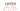### JS Coding Challenge: Find Anagrams

An anagram is a word or phrase formed by rearranging the letters of a different word or phrase, typically using all the original letters exactly once - from Wikipedia.

##### Challenge

Given an array of words, we need to write a function which will take two parameters. First parameter is a word and the second parameter is the array of the words. The function will return an array consisting of the anagrams of the word passed as the first parameter from the array of words passed as the second parameter.

###### Example:
```const words = ['mountain', 'anatomy', 'anemic', 'boldness', 'cinema', 'iceman', 'machine', 'mechanic', 'elbow', 'below', 'state', 'taste', 'dusty', 'night', 'study', 'thing', 'search', 'arches', 'chaser', 'animal', 'manila', 'icewoman']; const findAnagrams = (word, allWords) => { // Write the code here }; console.log(findAnagrams('cinema', words)); /* Expected output: ['anemic', 'iceman']; */```
###### Notes:
1. All the words in the returned result should have the same length as the given word. Example: iceman and icewoman are not anagrams. Even though iceman has every letter as in icewoman but icewoman has extra letters in it which iceman doesn't have.
2. The word passed as first parameter should not be included in the returned array. As in the code above you can see that cinema is not included in the expected output.
##### Algorithm
1. First, we need to find the total count of each letter in the word. Example: in cinema each letter has a total count of 1
2. Then, we need to loop through each word in the array of words and follow the Step 1 for each.
3. Then, we need compare the count of each letter between the given word and the current word in the iteration.
4. If the current word matches with the given word in terms of the letter and letter counts, we will push that word in the result array.
5. Follow Step 2 to Step 4 until the end of the words array
##### Solution

First, we will write a `helper` function which takes a word converted to an array of letters and will give back an `object` consisting of each letter in the word as the `keys` and the total counts of each letter as the `value`:

```const numberOfEachLetter = (letters) => { return letters.reduce((acc, letter) => ({ ...acc, [letter]: acc[letter] ? acc[letter] + 1 : 1, }), {}); };```

In the above function we are using `Array.reduce()` function to create an object of the letters and the count of each letter as the value. We are initiating the `.reduce()` function with an empty `object{}` which is provided as the second argument of the function.
And, in each iteration we are using the ES6 spread operator to get the previous value from and set updated value to `accumulator`. And then, using a `ternary` operator, we are checking if the current letter is already in the `accumulator` or not. If it is, then we are incrementing the count, otherwise we are setting 1 as the count value.

We can call the function like this:

```const word = 'cinema'; numberOfEachLetter(word.split('')); // Output { a: 1, c: 1, e: 1, i: 1, m: 1, n: 1 }```

Now, we will write another function which can compare between two words using the above `numberOfEachLetter` function:

```const hasSameLetterCount = (word1, word2) => { const word1Count = numberOfEachLetter(word1.split('')); const word2Count = numberOfEachLetter(word2.split('')); return word1.length == word2.length && Object.keys(word1Count) .every(letter => word1Count[letter] === word2Count[letter]); };```

Firstly, here we are getting the objects of letter counts for both words using the `hasSameLetterCount` function. Then, we are comparing the length of the two words to make sure that they have exact number of letters.
And finally, we are, using the `Object.keys()`, iterating through each letter of the first word and comparing to the letters of second word to check if the letters are same and have the same number of occurrence.
Using the `Array.every()` function we are checking that every letter and the count of the letters matches. Otherwise, the function will return `false`.

Okay, enough with the helper functions. Let's checkout the final function now:

```const findAnagrams = (word, allWords) => { const anagrams = allWords.filter(item => { return word !== item && hasSameLetterCount(word, item); }); return anagrams; };```

Here, using the `Array.filter()` function, we are iterating through each word in the words array and checking if the current word doesn't match with the given word and then sending the both words to the `hasSameLetterCount` function to check if they are matched to be anagrams. And finally returning the array of the filtered words that match with the criteria.

Does the final function look fat? Here is the slim version using the magic of ES6:

```const findAnagrams = (word, allWords) => allWords .filter(item => word !== item && hasSameLetterCount(word, item));```